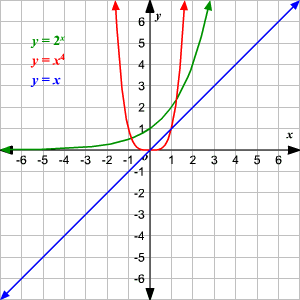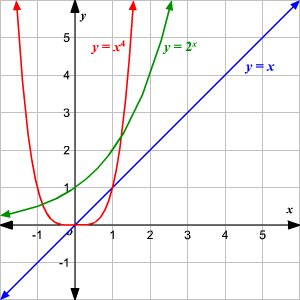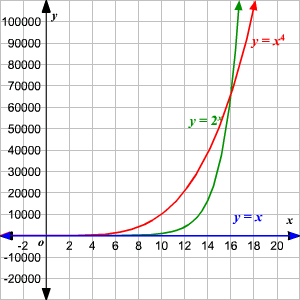# Comparing Linear, Polynomial, and Exponential Growth

Consider the three functions $y=x$ , $y={x}^{4}$ and $y={2}^{x}$ .The first is a linear function ; its graph is a straight line.

The second is a polynomial function .

The third is an exponential function .

Suppose you graph all three on the same axes, and ask the question, “Which function grows the fastest for large values of $x$ ?”

In this graph, it appears that the answer is $y={x}^{4}$ . It is greater than $y={2}^{x}$ for all values shown.However, if we zoom out the $y$ -axis quite a lot, we see that at $x=16$ , $y={2}^{x}$ overtakes $y={x}^{4}$ , and after this point, $y={2}^{x}$ grows faster. (Note that with the axes scaled this way, $y=x$ grows so slowly that it is indistinguishable from the $x$ -axis.)In fact, it can be shown that this is true for ANY exponential function and ANY polynomial function with positive growth. The exponential function will eventually outstrip the polynomial function.